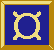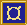# ¤ postProcessHigh, preProcessHigh, et al

## Types

```postProcessHigh :: SP a ho -> F hi a -> F hi ho
preProcessHigh :: F c ho -> SP hi c -> F hi ho
postProcessLow :: SP TCommand TCommand -> F hi ho -> F hi ho
preProcessLow :: F hi ho -> SP TEvent TEvent -> F hi ho
postMapHigh :: (a -> ho) -> F hi a -> F hi ho
preMapHigh :: F c ho -> (hi -> c) -> F hi ho
preMapLow :: F hi ho -> (TEvent -> TEvent) -> F hi ho
postMapLow :: (TCommand -> TCommand) -> F hi ho -> F hi ho
prepostMapHigh :: (hi -> b) -> (c -> ho) -> F b c -> F hi ho
prepostMapLow :: (TEvent -> TEvent) -> (TCommand -> TCommand) -> F hi ho -> F hi ho
>^^=< :: SP a b -> F e a -> F e b
>=^^< :: F c d -> SP e c -> F e d
>..=< :: SP TCommand TCommand -> F hi ho -> F hi ho
>=..< :: F hi ho -> SP TEvent TEvent -> F hi ho
>^=< :: (a -> b) -> F e a -> F e b
>=^< :: F c d -> (e -> c) -> F e d
>.=< :: (TCommand -> TCommand) -> F hi ho -> F hi ho
>=.< :: F hi ho -> (TEvent -> TEvent) -> F hi ho```

## Description

These functions are used to attach pre- and post-processors to fudgets.

There are two sets of operators. As suggested by the type signatures, the ones with `Map` (or a single `^` or `.`) as part of their names take functions that operate on single stream elements.

The operators with `Process` (or double `^^` or `..`) as part of their names take stream processors as arguments.

## Example

` (*2) >^=< fud   -- doubles the (numeric) output from fud`

## Equalities

The following equalities hold (except that the tagging of low level messages will be different). The left hand sides are likely to be more efficient.

```  sp  >^^=< fud  =  absF sp >==< fud
fud >=^^< sp   =  fud     >==< absF sp

f   >^=<  fud  =  mapF f  >==< fud
fud >=^<  f    =  fud     >==< mapF f
```